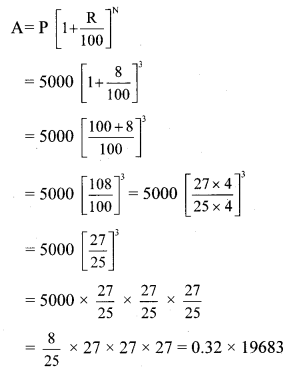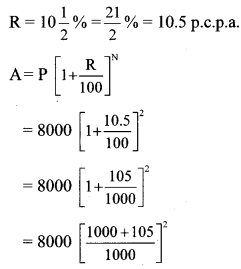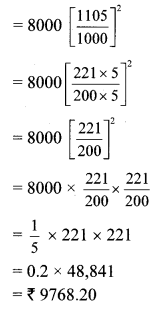# Maharashtra Board Class 8 Maths Solutions Chapter 14 Compound Interest Practice Set 14.1

## Maharashtra State Board Class 8 Maths Solutions Chapter 14 Compound Interest Practice Set 14.1

Practice Set 14.1 Class 8 Question 1.
Find the amount and the compound interest.

 No Principal (Rs) Rate (p.c.p.a.) Duration (years) i. 2000 5 2 ii. 5000 8 3 iii. 4000 7.5 2

Solution:
i. Here P = Rs 2000, R = 5 p.c.p.a. and N = 2 years= 5 × 441
∴ A = Rs 2205
I = Amount (A) – Principal (P)
= 2205 – 2000
= Rs 205
∴ The amount is Rs 2205 and the compound interest is Rs 205.

ii. Here, P = Rs 5000, R = 8 p.c.p.a. and N = 3 years∴ A = Rs 6298.56
I = Amount (A) – Principal (P)
= 6298.56 – 5000
= Rs 1298.56
∴ The amount is Rs 6298.56 and the compound interest is Rs 1298.56.

iii. Here, P = Rs 4000, R = 7.5 p.c.p.a. and N = 2 years∴A = Rs 4622.50
I = Amount (A) – Principal (P)
= 4622.50 – 4000
= Rs 622.50
∴The amount is Rs 4622.50 and the compound interest is Rs 622.50.

Compound Interest Practice Set 14.1 Question 2.
Sameerrao has taken a loan of Rs 12500 at the rate of 12 p.c.p.a. for 3 years. If the interest is compounded annually then how many rupees should he pay to clear his loan?
Solution:
Here, P = Rs 12,500, R = 12 p.c.p.a. and
N = 3 years= 0.8 × 28 × 28 × 28
= Rs 17,561.60
Sameerrao should pay Rs 17,561.60 to clear his loan.

8th Standard Maths Practice Set 14.1 Question 3.
To start a business Shalaka has taken a loan of Rs 8000 at a rate of $$10\frac { 1 }{ 2 }$$ p.c.p.a. After two years how much compound interest will she have to pay?
Solution:
Here, P = Rs 8000, N = 2 years andI = Amount (A) – Principal (P)
= 9768.20 – 8000
= Rs 1768.20
∴ After two years Shalaka will have to pay Rs 1768.20 as compound interest.